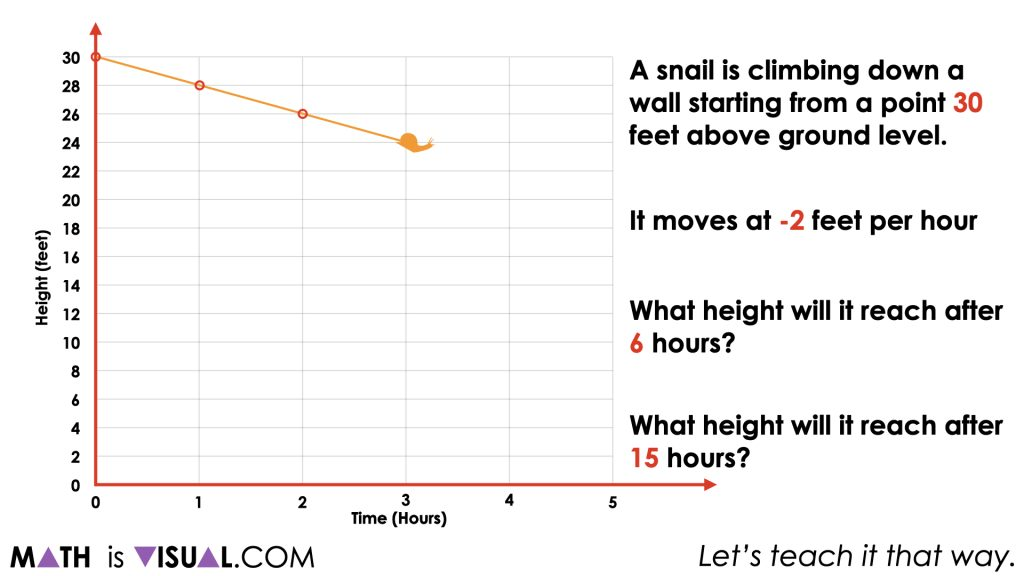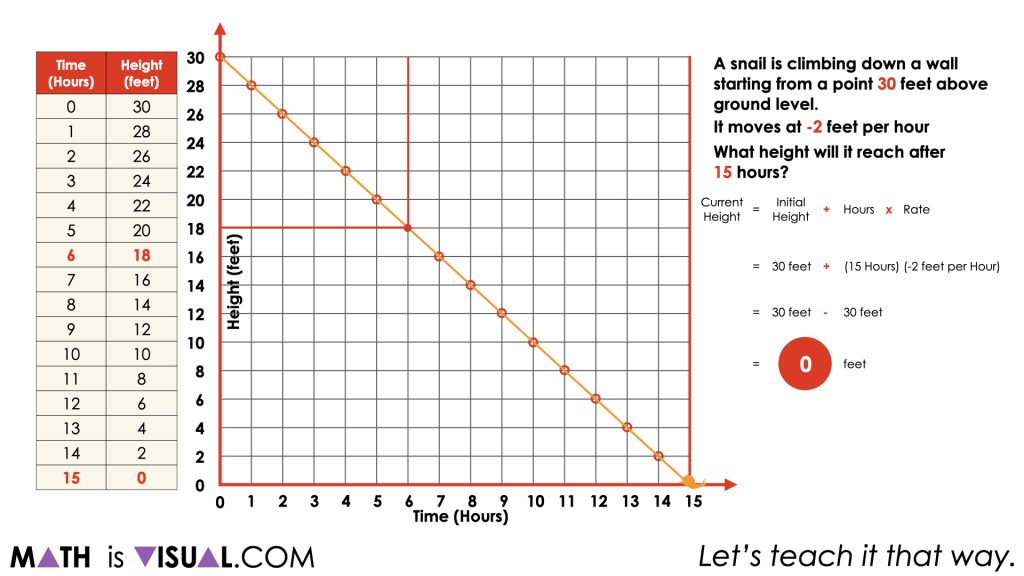# Multiplying Negative Integers Through

Investigate subtracting negative numbers by finding the difference in temperature using the thermometer as a linear model number line.

## In This Set of Visual Number Talk Prompts…

Students will explore integer subtraction through a real world contextual situation involving rising and falling temperatures.

## String of Related Problems

In today’s math talk, present the following graphical representations of patterns one at a time. For each pattern, ask students to create a context, describe the pattern rule in words, extend the pattern to 6 terms, and predict the value of the 15th term. Students are encouraged to turn and talk before sharing their responses with the larger group. When asked to determine the value of the 15th term, students should justify their answer.

## Visual Math Talk Prompt #1

Students will be prompted with:

A snail is climbing down a wall starting at a point 30 feet above ground level. It moves at a rate of -2 feet per hour. What height will it reach after 6 hours?

…15 hours?In this first visual number talk prompt, we are providing students with a positive initial value and a negative rate of change.

Allow students to leverage any models they feel most comfortable with to determine the height of the snail after 6 hours and 15 hours.

At this point, we are encouraging students to leverage tables, graphs and equations where possible. If a student is only leveraging one of the models, encourage them to represent using another model to add that tool to their repertoire.## Want to Explore These Concepts & Skills Further?

Three (2) additional number talk prompts are available in Day 6 of the Rising Water problem based math unit that you can dive into now.

Why not start from the beginning of this contextual 6-day unit of real world lessons from the Make Math Moments Problem Based Units page.

Did you use this in your classroom or at home? How’d it go? Post in the comments!

Math IS Visual. Let’s teach it that way.